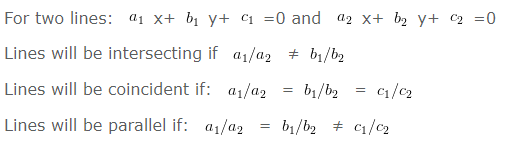# Pair of Linear Equations in Two Variables Class 10 Maths

Pair of Linear Equations in Two Variables Class 10 Maths, deals with various concepts which are as under:-

• Pair of Linear Equations in Two Variables
• Graphical Method of Solution of a Pair of Linear Equations
• Algebraic Methods of Solving a Pair of Linear Equations
Substitution Method
Elimination Method
Cross-Multiplication Method
• Equations Reducible to a Pair of Linear Equations in Two Variables

### 1. Linear Equations in two Variable

Equation having two variables i.e. x and y and where the maximum power of both the variable is 1 are called linear equation in two variables.

#### When are pair of lines intersecting, coincident or parallel